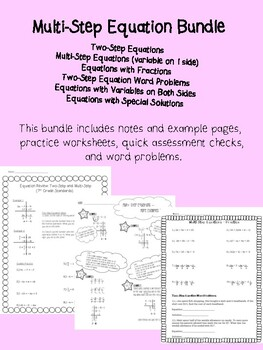# Multi-Step EquationsSubject
Resource Type
Common Core State Standards
Product Rating
4.0
1 Rating
File Type

PDF (Acrobat) Document File

1 MB|12 pages
Share
Product Description

MGSE8.EE.7b Solve linear equations with rational number coefficients, including equations whose solutions require expanding expressions using the distributive property and collecting like terms.

MGSE8.EE.7a Give examples of linear equations in one variable with one solution, infinitely many solutions, or no solutions. Show which of these possibilities is the case by successively transforming the given equation into simpler forms, until an equivalent equation of the form =, =, or = results (where and are different numbers).

This Bundle includes basic, easy-to-follow Examples, Notes, Guided Practice, Worksheets, Word Problems, and Quick Assessments (Ticket out the Door) on the following concepts:

Two-Step Equations

Multi-Step Equations (variable on 1 side)

Equations with Fractions

Two-Step Equation Word Problems

Equations with Variables on Both Sides

Equations with Special Solutions

Total Pages
12 pages
Not Included
Teaching Duration
N/A
Report this Resource to TpT
Reported resources will be reviewed by our team. Report this resource to let us know if this resource violates TpT’s content guidelines.